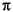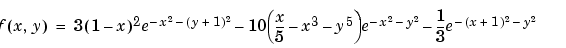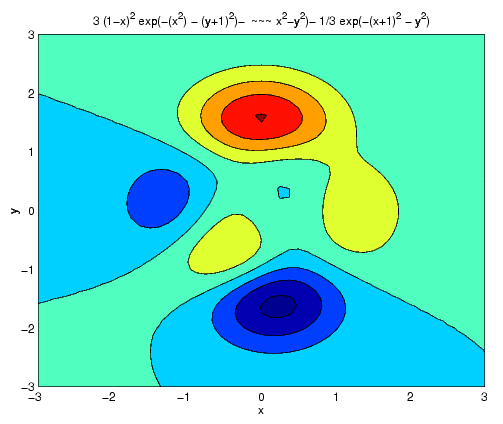MATLAB Function Referenceezcontourf

Easy to use filled contour plotter

Syntax

• ```ezcontourf(fun)
ezcontourf(fun,domain)
ezcontourf(...,n)
ezcontourf(axes_handle,...)
h = ezcontourf(...)
```

Description

`ezcontourf(fun)` plots the contour lines of `fun(x,y)`using the `contourf` function. `fun` is plotted over the default domain: -2< `x` < 2, -2< `y` < 2.

`fun` can be a function handle for an M-file function or an anonymous function (see Function Handles and Anonymous Functions) or a string (see the Remarks section).

```ezcontourf(fun,domain) ``` plots `fun(x,y)` over the specified `domain`. `domain` can be either a 4-by-1 vector [`xmin`, `xmax`, `ymin`, `ymax`] or a 2-by-1 vector [`min`, `max`] (where `min` < x < `max`, `min` < y < `max`).

```ezcontourf(...,n) ``` plots `fun` over the default domain using an `n`-by-`n` grid. The default value for `n` is 60.

```ezcontourf(axes_handle,...) ``` plots into the axes with handle `axes_handle` instead of the current axes (`gca`).

```h = ezcontourf(...) ``` returns the handles to contour objects in `h`.

`ezcontourf` automatically adds a title and axis labels.

Remarks

Passing the Function as a String

Array multiplication, division, and exponentiation are always implied in the string expression you pass to `ezcontourf`. For example, the MATLAB syntax for a filled contour plot of the expression

• ```sqrt(x.^2 + y.^2);
```

is written as

• ```ezcontourf('sqrt(x^2 + y^2)')
```

That is, `x^2` is interpreted as `x.^2` in the string you pass to `ezcontourf`.

If the function to be plotted is a function of the variables u and v (rather than x and y), then the domain endpoints `umin`, `umax`, `vmin`, and `vmax` are sorted alphabetically. Thus, `ezcontourf('u^2 - v^3',[0,1],[3,6])` plots the contour lines for u2 - v3 over 0 < u < 1, 3 < v < 6.

Passing a Function Handle

Function handle arguments must point to functions that use MATLAB syntax. For example, the following statements define an anonymous function and pass the function handle `fh` to `ezcontourf`.

• ```fh = @(x,y) sqrt(x.^2 + y.^2);
ezcontourf(fh)
```

Note that when using function handles, you must use the array power, array multiplication, and array division operators (`.^, .*, ./`) since `ezcontourf` does not alter the syntax, as in the case with string inputs.

Passing Additional Arguments

If your function has additional parameters, for example `k` in `myfun`:

• ```function z = myfun(x,y,k)
z = x.^k - y.^k - 1;
```

then you can use an anonymous function to specify that parameter:

• ```ezcontourf(@(x,y)myfun(x,y,2))
```

Examples

The following mathematical expression defines a function of two variables, x and y.

•`ezcontourf` requires a string argument that expresses this function using MATLAB syntax to represent exponents, natural logs, etc. This function is represented by the string

• ```f = ['3*(1-x)^2*exp(-(x^2)-(y+1)^2)',...
'- 10*(x/5 - x^3 - y^5)*exp(-x^2-y^2)',...
'- 1/3*exp(-(x+1)^2 - y^2)'];
```

For convenience, this string is written on three lines and concatenated into one string using square brackets.

Pass the string variable `f` to `ezcontourf` along with a domain ranging from -3 to 3 and specify a grid of 49-by-49:

• ```ezcontourf(f,[-3,3],49)```

In this particular case, the title is too long to fit at the top of the graph, so MATLAB abbreviates the string.

See Also

`contourf`, `ezcontour`, `ezmesh`, `ezmeshc`, `ezplot`, `ezplot3`, `ezpolar`, `ezsurf`, `ezsurfc`, `function_handle`

Contour Plots for related functions

© 1994-2005 The MathWorks, Inc.